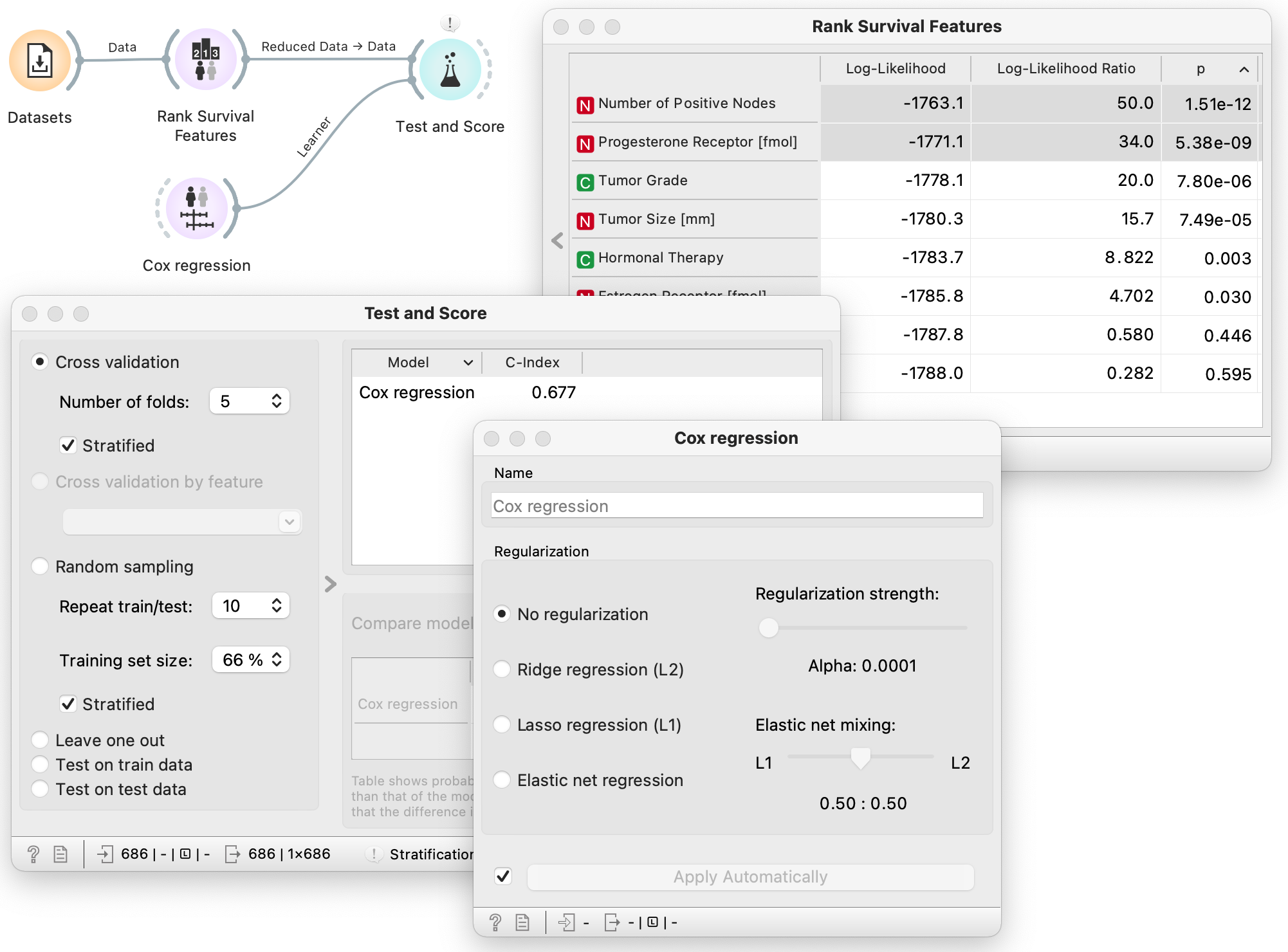# Cox Regression

Fit the Cox regression model on input data.

Inputs

• Data: reference survival dataset
• Preprocessor: preprocessing method

Outputs

• Learner: Cox regression learning algorithm
• Model trained model
• Coefficients: Cox regression coefficients

Cox Regression is a method for investigating the effect of several variables upon the time a specified event takes to happen. It assumes that the effects of the predictor variables upon survival are constant over time and are additive in one scale.

## Example

In this example we estimate the concordence index for the Cox regression model trained on data instances from selected features by using cross-validation. The concordance index quantifies the quality of rankings and is the standard performance measure for model assessment in survival analysis.We first load the German breast cancer study group 2 (German BC2) dataset using the widget Datasets. Next we input the data into the Rank Survival Features widget and select the top two most informative features, Number of Positive Nodes and Progesterone Receptor. We input this reduced dataset comprised only of the selected features and the Cox Regression learner into Test and Score, where we can inspect the cross-validated concordance measure.